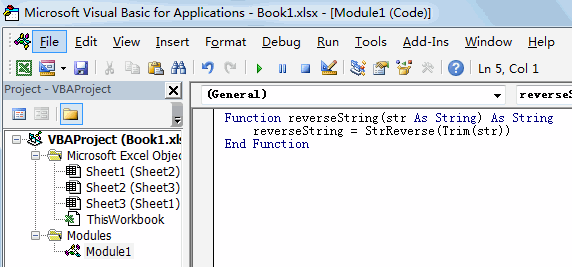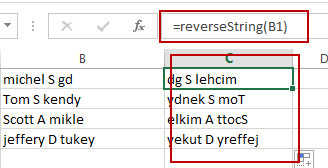# Reverse Text String in Excel

This post will guide you how to reverse text string in Excel. How do I reverse text string in one cell with an Excel User Defined Function. How to reverse a text string in one cell with a formula in Excel.

## Reverse Text String with User Defined Function

You can easily write down a User Defined Function to reverse a text string in one cell. Just do the following steps:

#1 open your excel workbook and then click on “Visual Basic” command under DEVELOPER Tab, or just press “ALT+F11” shortcut.#2 then the “Visual Basic Editor” window will appear.

#3 click “Insert” ->”Module” to create a new module.#4 paste the below VBA code into the code window. Then clicking “Save” button.Function reverseString(str As String) As String

reverseString = StrReverse(Trim(str))

End Function

#5 Type the following formula into blank cells and then press Enter key.

=reverseString(B1)## Reverse Text String with Formula

You can also use an Excel Array formula based on the TEXTJOIN function, the MID function, the ROW function, the INDIRECT function and the LEN function to reverse text string in one cell. Like this:

`=TEXTJOIN(“”,TRUE,MID(B2,LEN(B2)-ROW(INDIRECT(“1:”&LEN(\$B\$2)))+1,1))`

Type this formula into a blank cell, and then press Ctrl + Shift + Enter keys.

### Related Functions

• Excel TEXTJOIN function
The Excel TEXTJOIN function joins two or more text strings together and separated by a delimiter. you can select an entire range of cell references to be combined in excel 2016.The syntax of the TEXTJOIN function is as below:= TEXTJOIN  (delimiter, ignore_empty,text1,[text2])…
• Excel ROW function
The Excel ROW function returns the row number of a cell reference.The ROW function is a build-in function in Microsoft Excel and it is categorized as a Lookup and Reference Function.The syntax of the ROW function is as below:= ROW ([reference])….
• Excel MID function
The Excel MID function returns a substring from a text string at the position that you specify.The syntax of the MID function is as below:= MID (text, start_num, num_chars)…
• Excel INDIRECT function
The Excel INDIRECT function returns the cell reference based on a text string, such as: type the text string “A2” in B1 cell, it just a text string, so you can use INDIRECT function to convert text string as cell reference….